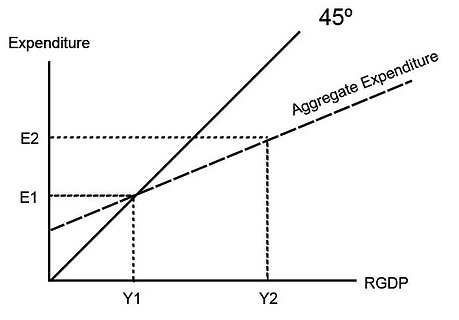Aggregate Expenditure Model

Aggregate Expenditure Model

ContributorsChristian Bien​What is the Aggregate Expenditure Model?The aggregate expenditure model is a model that measures the effect of an increase in one or more components of aggregate expenditure on real Gross Domestic Product.What does the Aggregate Expenditure Model Look Like?Multiplier ProcessWant your ATAR notes to empower over 77,000 students per year?Join the Team. Empower Education.Get instant access to all content and subscribe to our weekly email list on study tips, opportunities and other free resources.

It only takes a minute...• Expenditure (Y-Axis) This is used to show the effect of an initial change in one of more components of aggregate expenditure. E meaning the level of expenditure.

• Real GDP (X-Axis) This is used to show the multiplier effect and the greater change in RGDP from an initial change in Expenditure. - 45º Line The line shows where real GDP equals expenditure.​​​​​